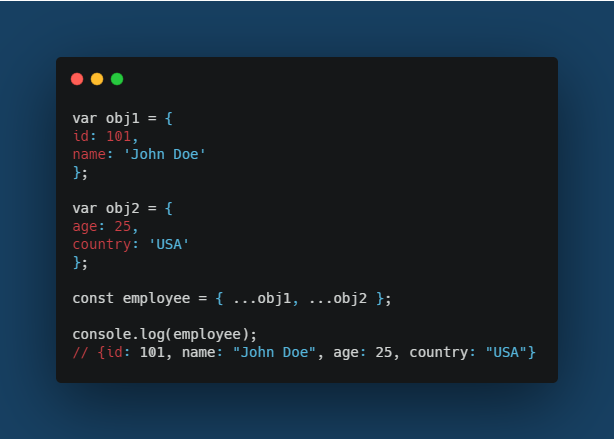# 5 Uses of the ES6 Spread OperatorES6 has not only made JavaScript more efficient but also more fun. Modern browsers all support the new ES6 syntax, so if you haven’t taken the time to play around, you definitely should.

The spread operator was introduced in ES6 (ECMAScript 6). It is basically three dots (…). It expands something into individual elements — hence the term spread.

Spread syntax allows an iterable such as an array expression or string to be expanded in places where zero or more arguments (for function calls) or elements (for array literals) are expected, or an object expression to be expanded in places where zero or more key-value pairs (for object literals) are expected.

I first got to know about spread operators when I was learning React. Since then, I’ve become a big fan of the spread operator. These three dots that may change the way you complete tasks within JavaScript.

The following is a listing of my favorite uses of the spread operator within JavaScript!

## Uses of the Spread Operator

### Copying an array

The traditional way was to use the `Array.prototype.slice()`method. But with the spread operator, it just takes three dots …

``````let names = ['John','James','Ben'];

let newNamesArray = [...names];

console.log(newNamesArray); // ['John','James','Ben']
``````

## Concatenating arrays

This objective was previously achieved by using the `Array.prototype.concat()` method. Now it can be easily achieved using the spread operator.

``````let arr1 = ['A', 'B', 'C'];

let arr2 = ['H', 'I', 'J'];

let result = [...arr1, ...arr2];

console.log(result); // ['A', 'B', 'C', 'H', 'I', 'J']
``````

## Spreading elements together with an individual element

This was kind of troublesome with an implementation like this.

``````// normally used expand method
let arr = ['a','b'];
let arr2 = [arr,'c','d'];
console.log(arr2); // [ [ 'a', 'b' ], 'c', 'd' ]
``````

As you can see, the array has been nested inside the parent array. This was an issue with the previous implementation. But with the spread operator, the intended outcome can be easily achieved.

``````let fruits = ['Apple','Pineapple','Banana'];

let newFruits = ['Mango', ...fruits];

console.log(newFruits); // ['Mango', 'Apple','Pineapple','Banana']
``````

## Spreading elements on function calls

As you can see in the below example, the three dots have made this task look quite simple. But if you try to do it manually, you would have to access and pass all the elements individually.

``````let names = ['John','James','Ben'];

var getNames = (f1, f2, f3) => {

console.log(`Names: \${f1}, \${f2} and \${f3}`);

};

getNames(...names); // Names: John, James and Ben
``````

## Spread syntax for object literals

The traditional way of achieving this was by using the `Object.assign()` method.

The `Object.assign()` method can be used to copy the values of all enumerables’ own properties from one or more source objects to a target object, and it will return the target object.

But with the spread operator, three dots simply get the job done.